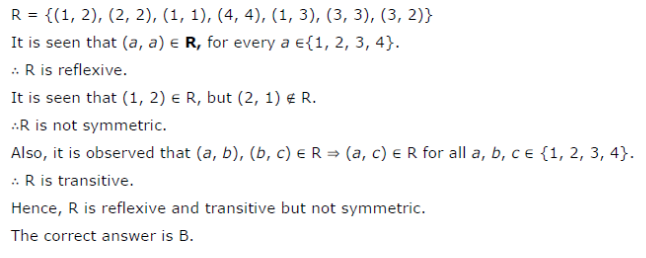# Let R be the relation in the set {1, 2, 3, 4} given by R = {(1, 2), (2, 2), (1, 1), (4, 4), (1, 3),

Let R be the relation in the set {1, 2, 3, 4} given by R = {(1, 2), (2, 2), (1, 1), (4, 4), (1, 3), (3, 3), (3, 2)}. Choose the correct answer:
(A) R is reflexive and symmetric but not transitive.
(B) R is reflexive and transitive but not symmetric.
( C) R is symmetric and transitive but not reflexive.
(D) R is an equivalence relation.